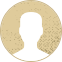Top Special Offer! Check discount

Get 13% off your first order - useTopStart13discount code now!

# Distance, Time, and Speed

183 views 2 pages ~ 501 words
Get a Custom Essay Writer Just For You!

Experts in this subject field are ready to write an original essay following your instructions to the dot!

a) Time taken for the signals to reach us after travelling through space is 14.6 hours.

1 minute is equivalent to 60 seconds and an hour has 60 minutes,

Therefore, the number of seconds in one hour can be calculated as 60 x 60 = 3600 seconds.

If                   1 hour has 3600 seconds,

\     14.6 hours will have: [14.6 x 3600]/1 = 52560 seconds

Therefore, there are approximately 52600 seconds in 14.6 hours hence the answer is 52600 seconds.

b) The distance between the Earth and Sun is 1Astronomical Unit (AU), which is also equivalent to 1.5 x 10^8 km.

The distance between a comet and the Earth is 73.7 AU.

If 1 AU = 150,000,000 km

\ 73.7 AU = (73.7 AU x 150,000,000 km)/1 AU

The result becomes 110.55 x 10^8 km

Therefore, the distance is 111 x 10^8 km

c) Distance between the space probe and the Earth is 2.54 x 10^9 km

If 1.5 x 10^8 km is equivalent to 1AU

\ 2.54 x 10^9 km = (2.54 x 10^9 km x 1AU)/ (1.5 x 10^8 km)

= 1.693 x 10^1 AU

= 16.93 AU

The answer to three significant figures is 16.9 AU

\ The distance between the space probe and the Earth is 16.9 AU

2. Time, Distance, and Speed

a) The speed of travel of radio waves is similar to that of light which is equivalent to 300,000 kilometers per second.

It takes 276 seconds for light to travel from the planet Venus to Earth.

If it takes 1 second for light to travel 300,000 km

\  Distance covered by the same light in 276 seconds is calculated as:

(276 seconds x 300,000 km)/ 1second = 82,800,000 km

= 8.28 x 10^7 km

\ Distance to Venus is 8.28 x 10^7 km

b) Time taken for light to reach us from the comet is 2.6 hours.

If 1 hour = 3600 seconds

\ 2.6 hours = (2.6 hours x 3600 seconds)/ 1 hour

= 9360 seconds

Radio waves travel at a speed of 300,000km/s in space.

So, if 1 second = 300,000km

9360 seconds = (9360 seconds x 300,000km)/1 second

= 2.808 x 10^9 km

The distance to this comet is therefore 2.808 x 10^9 km

Now if 1.5 x 10^8 km = 1 AU

\     2.808 x 10^9 km = (2.808 x 10^9 km x 1AU)/ (1.5 x 10^8 km)

= 18.72 AU

= 18.7 AU

Hence, the distance to this comet is 18.7 AU

c) Distance between space probe and the Earth is 25.4 AU

To convert this distance from kilometers to Astronomical Units:

Now 1 AU = 150,000,000 km

\ 25.4 AU = (25.4 AU x 150,000,000 km)/ 1 AU

= 3.81 x 10^9 km

Radio waves travel at a speed of 300,000km/s, therefore, it means that;

300,000 km = 1 second

To obtain the amount of time it takes for radio waves to travel from the space probe to Earth; (3.81 x 10^9 km x 1 second)/ 300,000 km,

which is equivalent to 1.27 x 10^4 seconds

Converting the time to hours:

If 3600 seconds = 1 hour

\ 1.27 x 10^4 seconds = (1 hour x 1.27 x 10^4 seconds)/ 3600 seconds

= 3.5278 hours

= 3.53 hours

Time taken for the radio waves to travel this distance is 3.53 hours

d) The space probe travels a distance of 17.6 AU in 6.23 years

Now, if 1 year has about 365.25 days

6.23 years will have; (365.25 days x 6.23 years)/ 1 = 2275.5075 days

If 1 hour has 3600 seconds, then 24 hours (1 day) have 3600 x 24 = 86400 seconds

Now that 1 day = 86400 seconds

2275.5075 days = (86400 seconds x 2275.5075 days)/ 1 day

= 1.96603848 x 10^8 seconds

Converting 17.6 AU to kilometers; (150,000 km x 17.6 AU)/ 1 AU = 2.64 x 10^9 km

Therefore, the space probe covers a distance of 2.64 x 10^9 km in 1.96603848 x 10^8 seconds.

Now if 1.96603848 x 10^8 seconds = 2.64 x 10^9 km

\                                       1 second = (1 second x 2.64 x 10^9 km)/ 1.96603848 x 10^8 seconds

= 1.34280180 x 10^1 km

Therefore, the average speed of the space probe is 13.4 km/s

August 04, 2023
Subcategory:
Subject area:
Number of pages

2

Number of words

501

41Rate:

4.3

Expertise Exploration
Verified writer

Working on a team project on global warming, I contacted PeterB. He is an excellent writer who will not only provide you with great sources but also fix all your grammar mistakes if you have a draft.

Hire Writer

This sample could have been used by your fellow student... Get your own unique essay on any topic and submit it by the deadline.

Eliminate the stress of Research and Writing!

Hire one of our experts to create a completely original paper even in 3 hours!